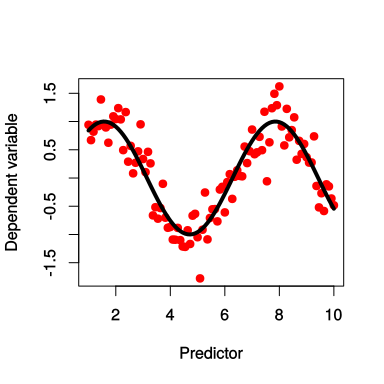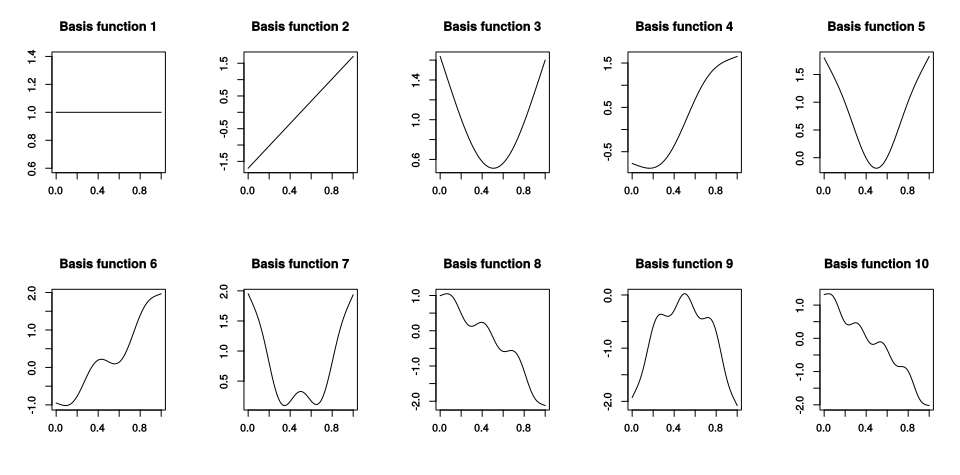# Generalized additive modeling

Martijn Wieling
University of Groningen

## This lecture

• Introduction
• Generalized additive modeling
• Articulography
• Using articulography to study L2 pronunciation differences
• Design
• Methods: R code
• Results
• Discussion

## Generalized additive modeling (1)

• Generalized additive model (GAM): relaxing assumption of linear relation between dependent variable and predictor
• Relationship between individual predictors and (possibly transformed) dependent variable is estimated by a non-linear smooth function: $$g(y) = s(x_1) +s(x_2,x_3) + \beta_4x_4 + ...$$
• Multiple predictors can be combined in a (hyper)surface smooth (other lecture)## Generalized additive modeling (2)

• Advantage of GAM over manual specification of non-linearities: the optimal shape of the non-linearity is determined automatically
• Appropriate degree of smoothness is automatically determined by minimizing combined error and "wigglyness" (no overfitting)
• Maximum number of basis functions limits the maximum amount of non-linearity

## First ten basis functions## Generalized additive modeling (3)

• Choosing a smoothing basis
• Single predictor or isotropic predictors: thin plate regression spline (this lecture)
• Efficient approximation of the optimal (thin plate) spline
• Combining non-isotropic predictors: tensor product spline
• Generalized Additive Mixed Modeling:
• Random effects can be treated as smooths as well (Wood, 2008)
• R: gam and bam (package mgcv)
• For more (mathematical) details, see Wood (2006) and Wood (2017)

## Articulography## Present study: goal and setup

• 19 native Dutch speakers from Groningen
• 22 native Standard Southern British English speakers from London
• Material: 10 minimal pairs [t]:[θ] repeated twice:
• 'tent'-'tenth', 'fate'-'faith', 'forth'-'fort', 'kit'-'kith', 'mitt'-'myth'
• 'tank'-'thank', 'team'-'theme', 'tick'-'thick', 'ties'-'thighs', 'tongs'-'thongs'
• Note that the sound [θ] does not exist in the Dutch language
• Goal: compare distinction between this sound contrast for both groups
• Preprocessing:
• Articulatory segmentation: gestural onset to offset (within /ə/ context)
• Positions $$z$$-transformed per axis and time normalized (from 0 to 1) per speaker